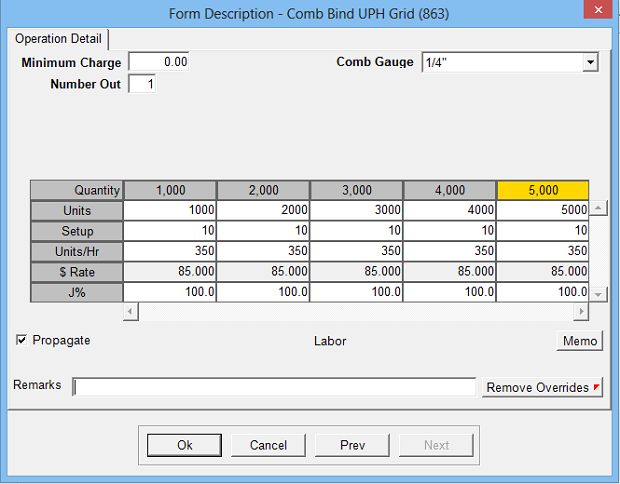Estimates the cost and time of an operation based on user defined column selection that varies setup minutes and speed with quantity breaks.

Operation Setup Requirements:
1. Description
2. Rate: Cost per hour
3. Sell \$ use: Markup % (recommended) or alternate sell rate
4. Calc: 25
5. Sub Key: Labor
6. Minimum Charge: Option to define minimum dollar charge
7. Unit of Measure: Unit
8. Column Label: Variable that estimator will select to choose corresponding specifications
9. Grid Quantity Column: Used to set quantity breaks for UPH adjustments
10. Column Headers: Used to describe column
11. Grid Data: Enter the setup minutes, rate and speed (for each quantity break) for each defined columnEstimate Specification Input Prompts:

1. Minimum Charge
2. Number Out
3. User defined pull-down menu (column selector)
4. Units
5. Setup Minutes
6. Units/Hr
7. J%
8. Remarks
9. MemoCalculation:
Units (if last bindery operation) = (Estimate Quantity / Number Out) x Idents
Units (if not last bindery operation) = ((Next Bindery Gross Qty + Next Bindery MR Qty) / Number Out) x Idents
Run Time = Units / UPH
Total Time = Run Time + Setup Time
Total Time x Rate \$ = Cost \$
Cost \$ x Markup % = Markup \$
Cost \$ + Markup \$ = Sell \$